We’d like to understand how you use our websites in order to improve them. Register your interest.

# Atmospheric neutrinos in the context of muon and neutrino radiography

## Abstract

Using the atmospheric neutrinos to probe the density profile of the Earth depends on knowing the angular distribution of the neutrinos at production and the neutrino cross section. This paper reviews the essential features of the angular distribution with emphasis on the relative contributions of pions, kaons and charm.

## 1. Introduction

In principle atmospheric neutrinos can be used to measure the density profile of the Earth (integrated along a chord) by comparing the observed rate of upward-moving neutrino-induced muons as a function of zenith angle with what is produced in the atmosphere. The measurement depends on several factors: the zenith angle dependence of neutrino production in the atmosphere, the differential neutrino cross section as a function of energy, properties of muon propagation in the medium surrounding the detector and the angular and energy resolution of the detector. Gonzalez-Garcia et al. (2008) show that it may be possible to measure some aspects of the core-mantle transition region with the IceCube detector currently under construction at the South Pole (Karle et al., 2007). A data accumulation of order ten years or more would be needed.

The critical energy range for such a measurement is 10 ≤ E v ≤ 100 TeV where the absorption of the Earth becomes important, first for neutrinos coming straight up through the diameter of the Earth, and at higher energy for larger angles. Hoshina (2008) discusses the angular resolution of IceCube for neutrino-induced muons from muon neutrinos in this energy band, where more than 50% of events can be reconstructed within one degree of their true direction. The charged current neutrino cross sections in this energy range are known to within ±2% (Cooper-Sarkar and Sarkar, 2008). In this paper, I focus on the angular distributions of muon neutrinos produced in the atmosphere and their uncertainties.

## 2. Angular Dependence of Atmospheric Neutrinos

When cosmic ray protons and nuclei enter the atmosphere they interact and produce cascades of secondaries, including charged hadrons, some of which decay to produce muons and neutrinos. Muon neutrinos of high energy are produced primarily in the decay chain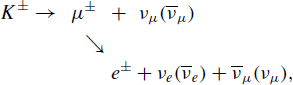(1)

There are similar expressions for decay chains initiated by pions and charmed hadrons. In the energy range of interest here, decay of muons is rare and its contribution to muon neutrinos can be neglected. Moreover, the contribution from kaons is large (80%) as compared to the contribution from decay of charged pions (20%). The contribution from charm decay is small, but may become significant in the energy region of interest here, as discussed below.

Neutrino fluxes are determined by a combination of four factors:

1. 1)

the energy spectrum and composition of the primary cosmic radiation,

2. 2)

production of pions, kaons and charmed hadrons in collisions of nucleons and other hadrons with nuclei of the atmosphere,

3. 3)

kinematics of hadron decays to neutrinos, and

4. 4)

the density profile of the atmosphere.

The primary spectrum can be approximated by a sequence of power laws. The energy range relevant for 10–100 TeV neutrinos is of order 30 TeV to 3 PeV, where the spectrum of nucleons is approximately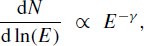(2)

with γ ≈ 1.7 (Gaisser and Stanev, 2008).

For each of the main neutrino parents (i), there is a critical energy,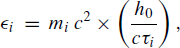(3)

where h0 is the scale height of an exponential approximation to the atmosphere and m i and τ i are respectively the mass and rest lifetime of a particle of type i = π±, K±, D±. When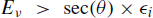(4)

re-interaction of the parent hadron is favored over its decay. Approximate numerical values are given in Table 1.

The energy dependence of the competition between decay and re-interaction of the different parent mesons affects the neutrino energy spectrum in an essential way. These effects can be displayed explicitly using analytic approximations for the neutrino energy spectrum. In the power-law approximation and at high energy, the spectrum of neutrinos, φ v , is approximated well by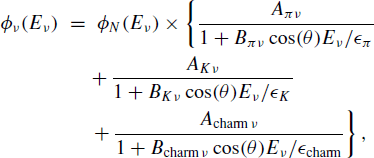(5)

where φ N (E v ) = dN/d ln(E v ) is the primary spectrum of nucleons (N) evaluated at the energy of the neutrino (Gaisser, 1990). The factors A iv contain the physics of meson production weighted by the spectrum and the decay kinematics contained in Eq. (1).

As an example,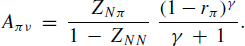Here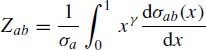is the spectrum weighted moment for the interaction process in which a particle a interacts with a nucleus in the atmosphere and produces a secondary particle b that has a fraction x of the lab energy of the projectile. The factor involving r π = m2 π is the spectrum weighted kinematic factor for the decay πμ + v and γ ≈ 1.7 is the integral spectral index in a power-law approximation to the primary cosmic ray spectrum.

At low energy, the neutrino spectrum follows the same power law as the parent hadrons. At high energy, the probability of decay of a charged pion or kaon is suppressed relative to hadronic interaction by a factor proportional to the energy of the meson. This suppression is represented by the denominator of each term in Eq. (5). Asymptotically at high energy the neutrino spectrum has an extra factor of 1/E relative to the spectrum at low energy, which is proportional to the primary cosmic-ray spectrum. This steepening of the neutrino spectrum occurs at higher energy for larger zenith angles as a consequence of the sec(θ) factor, which occurs because particle production occurs higher in less dense atmosphere for large angles. The factors B iv in the denominators are ratios of spectrum-weighted kinematic factors for meson decay to neutrinos to account for the fact that the decay occurs from a steeper spectrum at higher energy. Close to the horizontal (θ > 70°) the curvature of the Earth is significant and the secant is to be evaluated at the local zenith angle where the particle production occurs, which is less than the zenith angle of the trajectory at the detector (Lipari, 1993).

Parameterizations of the form of Eq. (5) represent detailed numerical and Monte Carlo calculations rather well. The corresponding parametric equations for muons can be compared directly to the many measurements of atmospheric muons. Such a comparison is made in figure 24.4 Gaisser and Stanev (2008), for example. (See also figure 4 of Gaisser (2005).)

Uncertainties in the angular dependence arise primarily from uncertainties in the level of kaon production for E v 10 TeV and at higher energy from the more uncertain level of charm production. Ideally one would simply measure the downward atmospheric neutrino flux and compare it with the upward neutrino flux from the opposite direction and use the ratio Earth-in to Earth-out to measure the density profile in a way that is independent of the intrinsic angular dependence of the atmospheric neutrino beam. In reality this unfortunately cannot be done because the relatively high intensity of downward atmospheric muons hides the downward atmospheric neutrinos. Because of the close genetic relation between v μ and μ of Eq. (1) and the corresponding equation for pions, a measurement of downward atmospheric muons provides some control of the angular dependence. This constraint, while valid and worth pursuing, is of limited practical use in this context because of the fact that most muons come from decay of charged pions while, in the high energy range of interest here, most neutrinos come from decay of kaons.

## 3. Neutrinos from Kaons

Each term in Eq. (5) exhibits the same characteristic dependence on energy and zenith angle. Kaons become increasingly important with increasing energy because єK > є π . This means that the contribution from kaons retains a spectrum close to that of the primary cosmic rays while the pion contribution is already beginning to steepen. The location of this transition depends on the K/π ratio at production and is of the order of 100 GeV, as shown in Fig. 1. It occurs at higher energy for larger zenith angle. Note that kaons never become the dominant source of atmospheric muons. This is largely a consequence of the fact that the muon mass is not much less than its parent pion so the v μ carries only a small fraction of the energy in πμv μ .

Uncertainties in the calculated intensity of atmospheric neutrinos at high energy due to uncertain knowledge of hadronic interactions were estimated by Agrawal et al. (1996). The single largest source of uncertainty in the TeV range and above is from the uncertainty in kaon production, in particular in the factor ZpK+. This uncertainty was estimated as ±13% in the 10–100 TeV range.

The recent measurement of the μ+ / μ ratio above 1 TeV with the MINOS far detector (Adamson et al., 2007) reflects the importance of kaon production in an interesting way. The charge ratio increases as kaons become dominant, from which one can infer that the K+ / K ratio is larger than the π+ / π ratio. It is likely that the specific process of associated production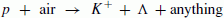(6)

plays an important role here. Adamson et al. have shown how their measurement can constrain the parameters that describe the production of kaons relative to pions and their charge ratios. Because of the genetic relation between atmospheric μ and v μ , the MINOS measurement, in combination with measurements of K / π production at high energy, has the potential also to make the prediction of the angular distribution of atmospheric muon neutrinos more precise.

## 4. Neutrinos from Charm

Although the charm channel for atmospheric neutrinos is formally the same as for pions and kaons, there are two large quantitative differences. One is that production of charm in hadronic interactions is very much smaller than production of pions and kaons. The other is that the critical energy is so high (because of the short charm lifetime) that muons and neutrinos from decay of charmed hadrons will continue in the low-energy regime of Eq. (5) with the same, relatively hard, spectrum as the primary cosmic-ray nucleons and with an isotropic angular distribution.

Because of its harder spectrum, the charmed contribution will eventually become the dominant source of atmospheric v μ , adding an isotropic “prompt” component to the secant(θ) dependence of the kaon and pion contributions. The transition energy is at some energy E v > 10 TeV, which therefore may be in the energy range relevant for Earth tomography with neutrinos. Because the level of charm production is so uncertain, this is a significant source of uncertainty in the angular distribution of the atmospheric neutrino beam in this high energy range. Gelmini et al. (2003) assemble constraints from measurements of the angular and energy distributions of atmospheric muons on charm production. An example of a model for charm production that is near the upper limit is the RQPM model of Bugaev et al. (1998). Figure 2 shows the angular distribution in the energy range 10 < E v < 20 TeV using this model. The prompt and normal components are shown separately.

In the model of Bugaev et al. atmospheric neutrinos from charm decay become equal in intensity to neutrinos from decay of kaons and pions at approximately 100 TeV. A more recent calculation (Enberg et al., 2008) predicts a level of charm production an order of magnitude lower than the model of Bugaev et al. In this case, the crossover occurs about a factor of two higher in energy.

## 5. Conclusion: Detecting Neutrinos

The neutrino effective area is defined so that the product of neutrino intensity multiplied by the effective area gives the event rate. The probability that a muon neutrino on a trajectory that will intercept the detector gives a visible muon in the detector is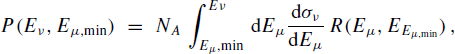(7)

where the integrand is the product of the charged current differential cross section and R is the average distance traveled by a muon with energy E μ at production before its energy is reduced to Eμ,min, the minimum energy required upon entering the detector for the event to be reconstructed well. The additional contribution from neutrinos that interact within a large detector can be computed in a straightforward way and added as an extra contribution.

The neutrino effective area is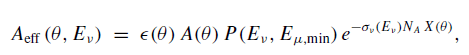(8)

where є(θ) is the efficiency for a detector of projected area A(θ) to detect a muon incident at zenith angle θ. The exponential expresses the muon attenuation in the Earth for angle θ below the horizon, where X(θ) is the amount of matter (g/cm2) along the chord through the Earth. Figure 3 shows Aeff for an idealized spherical detector with physical projected area of one km2 for several different zenith angles. The shaded region indicates the energy range of interest between 10 and 100 TeV. Because the neutrino spectrum decreases strongly with increasing energy, most of the rate will be near the lower end of this range. In Fig. 2, the prompt contribution is about 40% of the normal π, K channel contribution to the intensity of v μ near cos(θ) ≈ −0.9, which roughly corresponds to the core region. Because the level of charm is uncertain, this source of uncertainty in the angular distribution of v μ will be a significant limitation to the measurement of the core-mantle transition. Alternatively, a careful measurement of the angular distribution of atmospheric neutrino induced muons with good energy resolution in this energy range may clarify the level of charm production.

## References

1. Adamson, P. et al., MINOS Collaboration, Measurement of the atmospheric muon charge ratio at TeV energies with the MINOS detector, Phys. Rev. D, 76, 072005, 2007.

2. Agrawal, V., T. K. Gaisser, P. Lipari, and T. Stanev, Atmospheric neutrino flux above 1 GeV, Phys. Rev. D, 53, 1314, 1996.

3. Bugaev, E. V., A. Misaki, V. A. Naumov, T. S. Sinegovskaya, S. I. Sinegovsky, and N. Takahashi, Atmospheric muon flux at sea level, underground, and underwater, Phys. Rev. D, 58, 054001, 1998.

4. Cooper-Sarkar, A. and S. Sarkar, Predictions for high energy neutrino cross-sections from the ZEUS global PDF fits, JHEP, 1, 075, 2008.

5. Enberg, R., M. H. Reno, and I. Sarcevic, High energy neutrinos from charm in astrophysical sources, Phys. Rev. D, 79, 053006, 2008.

6. Gaisser, T. K., Cosmic Rays and Particle Physics, Cambridge University Press, 1990 (Japanese edition with K. Kobayakawa, Maruzen, 1997).

7. Gaisser, T. K., Outstanding problems in particle astrophysics, in Neutrinos and Explosive Events in the Universe, edited by M. M. Shapiro, T. Stanev, and J. P. Wefel, NATO Science Series II, Mathematics, Physics and Chemistry, Vo. 209, Springer, astro-ph/0501195, 2005.

8. Gaisser, T. K. and Todor Stanev, Cosmic Rays, in Reviews of Particle Properties, edited by C. Amsler, M. Doser et al., (Particle Data Group), Phys. Lett. B, 667, 254–260, 2008.

9. Gelmini, G., P. Gondolo, and G. Varieschi, Phys. Rev. D, 67, 017301, 2003.

10. Gonzalez-Garcia, M. C., F. Halzen, M. Maltoni, and H. K. M. Tanaka, Radiography of the Earth’s core and mantle with atmospheric neutrinos, Phys. Rev. Lett., 100, 061802, 2008.

11. Hoshina, K. for the IceCube Collaboration, these proceedings.

12. Karle, A. for the IceCube Collaboration, IceCube—construction, status, performance results of the 22 string detector, in Proc. 30th International Cosmic Ray Conference (Merida), 7–10, arXiv:0711.0353v1, 2007.

13. Lipari, P., Lepton spectra in the earth’s atmosphere, Astropart. Phys., 1, 195–227, 1993.

## Author information

Authors

### Corresponding author

Correspondence to Thomas K. Gaisser.

## Rights and permissions

Reprints and Permissions

Gaisser, T.K. Atmospheric neutrinos in the context of muon and neutrino radiography. Earth Planet Sp 62, 195–199 (2010). https://doi.org/10.5047/eps.2009.06.007

• Revised:

• Accepted:

• Published:

• Issue Date:

### Key words

• Neutrinos
• charm
• cosmic rays